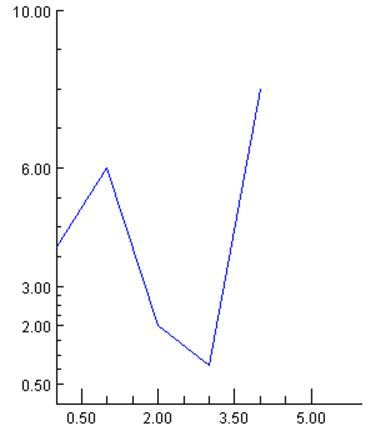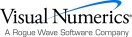## Major and Minor Tick Marks

The nodes MajorTick and MinorTick control the drawing of tick marks on an AxisXY.

The location of the major tick marks can be set explicitly, using the Axis1D.SetTicks method. However, it is usually easier to allow autoscaling to automatically set the tick locations (see Autoscale).

#### Attribute TickLength

The length of the tick marks is proportional to the screen size. They can be made relatively longer or shorter by setting the attribute TickLength. IMSL charting is largely made up of classes that inherit from ChartNode. Therefore AbstractChartNode.TickLength should be used to set the tick length. Its default value is 1.0. If its value is negative, the tick marks are drawn in the opposite direction: i.e., into the center of the plot region rather than away from it.

#### Attribute Number

Number is the number of major tick marks along an axis. IMSL charting is largely made up of classes that inherit from ChartNode. Therefore AbstractChartNode.Number should be used to set the number of ticks.

#### Attribute Density

Density is the number of minor tick marks in each interval between major tick marks. The minor ticks are equally spaced in user coordinates. If the Transform attribute in not TRANSFORM_LINEAR, then they will not be equally spaced on the screen. IMSL charting is largely made up of classes that inherit from ChartNode. Therefore AbstractChartNode.Density should be used to set the Density.

#### Attribute FirstTick

The FirstTick attribute, in an Axis1D node, is the position of the first major tick mark. Axis1D.FirstTick should be used to set the location of the first tick mark. The default value is the 0-th element of the Windows attribute.

#### Attribute TickInterval

The TickInterval attribute, in an Axis1D node, is the interval between tick marks in the user coordinates. “Axis1D.TickInterval should be used to set the tick interval. If this attribute is not explicitly set, its value is computed from the attributes Number, Window and Transform.

#### Attribute Ticks

The Ticks attribute, in an Axis1D node, contains the position of the major tick marks. Axis1D.SetTicks should be used to set the ticks. If this attribute is not explicitly set, its value is computed from the attributes FirstTick, TickInterval, Number, Window and Transform.

#### Example

This example shows the effects of the tick mark attributes. Note that autoscaling is turned off so that the attribute values are not overridden (see Autoscale).

On the x-axis, there are four major tick marks (Number), starting with 0.5 (First­Tick) at an interval of 1.5 (TickInterval). There are three minor tick intervals (Density) between each major tick mark. The tick marks are twice as long and are drawn in the opposite direction as normal (TickLength).

On the y-axis, the tick mark locations are set explicitly by the attribute Ticks. This automatically sets the attribute Number. The TickLength is set to -1, so the tick marks are drawn inward (to the right) instead of outward (to the left).using Imsl.Chart2D;

using System.Drawing;

public class SampleTicks : FrameChart {

public SampleTicks() {

Chart chart = this.Chart;

AxisXY axis = new AxisXY(chart);

axis.AutoscaleOutput = Axis.AUTOSCALE_OFF;

axis.AxisX.SetWindow(0.0, 6.0);

axis.AxisX.Density = 3;

axis.AxisX.Number = 4;

axis.AxisX.FirstTick = 0.5;

axis.AxisX.TickInterval = 1.5;

axis.AxisX.TickLength = -2;

axis.AxisY.SetWindow(0.0, 10.0);

double[] ticksY = {0.5, 2.0, 3.0, 6.0, 10.0};

axis.AxisY.SetTicks(ticksY);

axis.AxisY.TickLength = -1;

double[] y = {4, 6, 2, 1, 8};

Data data = new Data(axis, y);

data.DataType = Data.DATA_TYPE_LINE;

data.LineColor = Color.Blue;

}

public static void Main(string[] argv) {

System.Windows.Forms.Application.Run(new SampleTicks());

}

}Visual Numerics - Developers of IMSL and PV-WAVEhttp://www.vni.com/PHONE: 713.784.3131FAX:713.781.9260